# Numerical Analysis of the Parameters Governing 3D Laminar Mixed Convection Flow in a Rectangular Channel with Imposed Wall Flux Density

Numerical Analysis of the Parameters Governing 3D Laminar Mixed Convection Flow in a Rectangular Channel with Imposed Wall Flux Density

Lakhdar AidaouiYahia Lasbet Khaled Loubar

Laboratoire de Développement en Mécanique et Matériaux (LDMM), University of Djelfa, Djelfa 17000, Algeria

Ecole des Mines de Nantes. 44307 Nantes Cedex 3, France

Corresponding Author Email:
laidaoui@gmail.com
Page:
581-589
|
DOI:
https://doi.org/10.18280/ijht.340405
|
Accepted:
|
Published:
31 December 2016
| Citation

OPEN ACCESS

Abstract:

A numerical study is carried out in order to understand the effect of the parameters governing three dimensional (3D) laminar mixed convection flows in a rectangular channel with imposed wall flux density. The mixed convection can be governed and influenced by many factors which appear in the conservation equations. In the literature, no unanimity is found on the formulation of these parameters which can affect the bouncy forces in the motion equation, and consequently the onset of the mixed convection regime, the Nusselt number and the Poiseuille number. The principal result of this paper illustrates that the main factor characterizing the heat and flow regime of the mixed convection is the ratio between the Grashof number Gr, and the Reynolds number Re instead of the Richardson number Gr/Re2. Obtained results demonstrate as well the influence of the buoyancy parameter and Richardson number on the magnitude of the distortion of the flow velocity and therefore the flow structure.

Keywords:

Mixed convection, Rectangular channel, Nusselt number, Buoyancy parameter, Laminar flow.

1. Introduction

Convection is one of the heat transfer modes in addition to conduction and radiation, this transfer type can be arises between solid and flowing fluid. Heat transfer convection is divided into: forced convection caused by external forces like pumps and fans, and natural (free) convection when the motion is due only to the temperature difference between the wall and the fluid. The combination phenomenon between the two kinds: forced and free convections, is known as mixed convection, which occurs when the two convection mechanisms contribute concurrently together to the heat transfer. Mixed convection can be found in many industrial processes and engineering devices, such as: cooling of electronic devices, ventilation of premises, heat losses in the solar collectors, nuclear reactors cooling, optimisation of heat exchangers design and so on [1-2-3-4]. It is well known that several parameters can be affect mixed convection heat transfer and fluid flow within channels, such as: the Richardson number (Gr/Re2), the report of Grashof and Reynolds numbers (Gr/Re), the aspect ratio of the channel, reference temperature, etc. In the last decade, an increasing number of numerical and experimental researches processed the mixed convection phenomenon within different channel types wherein various parameters were used for characterising the heat and flow regime of the mixed convection. In some cases, the ratio between the Grashof number Gr and the Reynolds number Re (Gr/Re) for the onset was used as main parameter, in which it characterised the mixed convection regime in straight ducts. The corresponding values of the Gr/Re ratio are well determined in the literature, in addition to the kinematic of the flow, as in Elliot et al. , where mixed convection within a horizontal duct with constant wall temperature is studied numerically. Moreover, authors reported the dependency of the buoyancy driven fluid motion in the vertical direction on the Grashof and Reynolds numbers as well as the ratio of the duct height to its width. In Barletta et al. , the Gr/Re threshold values were evaluated through an analytical study of the mixed convection in vertical rectangular duct with a prescribed uniform wall heat flux. Besides, the effect of Gr/Re and the aspect ratio on the buoyancy and the Nusselt number were illustrated. Mohammed et al.  predicted numerically the nanofluids flow and heat transfer in an equilateral triangular duct, the obtained results revealed that the Nusselt number increases as Rayleigh number increases due to the buoyancy force effect. Yang et al. [8-9], defined the Gr/Re ratio as a buoyancy parameter assisted mixed convection flow and heat transfer. In their first work, they performed a numerical investigation of the laminar buoyancy assisted mixed convection and entropy generation in the entrance region of a vertical rectangular duct. In the second paper they study numerically the mixed convection flow in a symmetrically heated channel, with imposed inlet flow rate and uniform wall temperature. The obtained results permeated to understand the effect of aspect ratio and assisted buoyancy on flow and heat transfer characteristics.

From other hand, several researchers analysed the heat transfer characteristics of the mixed convection flow according to the Richardson number Gr/Re2. For example, when the heat transfer rate of mixed convection flow within a horizontal rectangular channel is investigated experimentally by Kurtbas et al. , the local and average Nusselt numbers are presented as functions of the Reynolds and Richardson numbers, in addition to the channel aspect ratio. Yang, M.H et al.  performed a numerical study of the mixed convection heat transfer in an inclined parallel-plate channel with a transverse fin placed in a chosen wall. The effects of the channel inclination angle, Richardson number and Reynolds number on the fin’s optimum aspect ratio and therefore its maximum heat transfer rate were discussed. Fu et al.  showed numerical results of flow reversal in mixed convection regime within 3D rectangular channel. Natural convection domination in the flow and thermal fields of the mixed convection in addition to the flow reversal phenomenon were discussed depending on the Richardson number. In a recent numerical study, Fu et al.  investigated the enhancement of heat transfer rate of mixed convection in a channel by an adjustable inlet boundary, during this study the Richardson number is used for comparison with previous studies. Marroquín-Desentis et al.  studied numerically the effects of buoyancy and channel inclination on transient laminar opposing mixed convection in rectangular channels with symmetric and discrete heating. The Richardson number parameter was used during this study in order to illustrate the buoyancy effect on the flow structure and the heat transfer (Nusselt number) from the heated slabs. Numerical simulations showed also the channel inclination angle effect on the flow and heat flux behaviour.

The reference temperature T0 used in the boussinesq approximation (equation 1) is also an important parameter in the modelling of buoyancy forces appeared in the motion equation and it is employed under different consideration.  As in Ichimiya et al. , Kurtbas et al. , Fakour et al.  and Yang et al. , where it is defined as the temperature of the channel inlet. While in Barletta et al. [18-19-6], the reference temperature for the mixed convection heat transfer was the mean fluid temperature in a duct section. A third choice of the reference temperature can be found in the literature [20-21], where the reference temperature is presented as a function of the Biot number and the channel walls temperatures.

The previous literature review reveals that a variety of parameters were used to characterize mixed convection heat transfer. As a consequence, a deep analysis of those parameters effects on the combined free and forced convection phenomenon within ducts is required.

In the present study, analysis of the parameters governing 3D laminar mixed convection flow in a rectangular channel with imposed constant wall flux density is investigated numerically. The commercial CFD (Computational fluid dynamics) code Fluent is employed to simulate the mixed convection heat flux in rectangular duct, besides the effects of the mentioned parameters (Gr/Re, Gr/Re2) on the heat transfer rate presented in terms of Nusselt number and flow reversal phenomenon will be examined.

2. Problem Description and Governing Equations

Figure 1 presents the physical model considered in the current study. Fluid flow within a three-dimensional vertical and rectangular channel with square cross section (1 cm x 1 cm) and an infinite length along the z coordinate (N periods, with each period equal to 10 cm) was analysed. Newtonian fluid (air) with constant temperature and fully developed velocity profile for rectangular ducts flows steadily from the channel bottom along the z direction. In this numerical study the four walls of the duct are maintained to a constant wall flux density q0. In the physical model (figure 1) the origin of coordinates is fixed at the centre of the channel entrance. The vector of the acceleration gravity is parallel and opposite in direction to z-axis.

## 1.jpg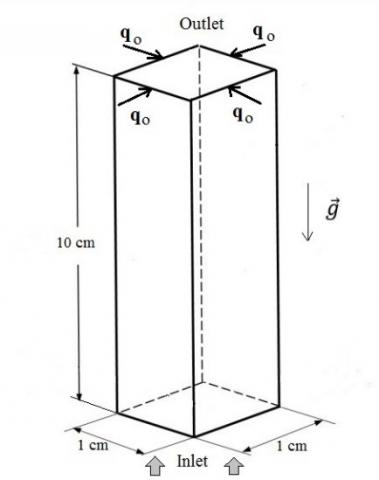Figure 1. Schematics of the physical model

In this work, the flow regime is considered laminar, the effect of viscous dissipation in the fluid is neglected and the physical properties of the fluid (dynamic viscosity, thermal conductivity and thermal diffusivity) are supposed to be constants, except the fluid density in the buoyancy parameter, in which the Boussinesq approximation is used and presented by the equation of state:

$\rho=\rho_{0}\left[1-\beta\left(T-T_{0}\right)\right]$(1)

In the current study, the reference temperature T0 is identical to the inlet cross section temperature.

The dimensionless form of the governing equations of continuity (2), momentum (3) and energy (4) in three dimensions are as follow:

$\frac{\partial U_{x}}{\partial X}+\frac{\partial U_{y}}{\partial Y}+\frac{\partial U_{z}}{\partial z}=0$(2)

$U_{x} \frac{\partial U_{x}}{\partial X}+U_{y} \frac{\partial U_{x}}{\partial Y}+U_{z} \frac{\partial U_{x}}{\partial Z}=-\frac{\partial P}{\partial X}+\frac{1}{R e} \nabla^{2} U_{x}$

$U_{x} \frac{\partial U_{y}}{\partial X}+U_{y} \frac{\partial U_{y}}{\partial Y}+U_{z} \frac{\partial U_{y}}{\partial z}=-\frac{\partial P}{\partial Y}+\frac{1}{R e} \nabla^{2} U_{y}$(3)

$U_{x} \frac{\partial U_{z}}{\partial X}+U_{y} \frac{\partial U_{z}}{\partial Y}+U_{z} \frac{\partial U_{z}}{\partial Z}=-\frac{\partial P}{\partial Z}+\frac{1}{R e} \nabla^{2} U_{z}+\frac{G r}{R e^{2}} \theta$

$U_{x} \frac{\partial \theta}{\partial X}+U_{y} \frac{\partial \theta}{\partial Y}+U_{z} \frac{\partial \theta}{\partial z}=\frac{1}{R e P r} \nabla^{2} \theta$(4)

In which the dimensionless variables are defined as:

$\vec{U}=\frac{\vec{u}}{u_{z, 0}}, X=\frac{x}{a}, Y=\frac{y}{a}, Z=\frac{z}{a}, P=\frac{p}{\rho u_{z, 0}^{2}}, \theta=\frac{T-T_{\infty}}{\Delta T}$

The dimensionless parameters used in the previous formulations are Reynolds number, Grashof number and Prandtl number respectively:

$R e=\frac{u_{z, 0} D}{v}, G r=\frac{g \beta \Delta T D^{3}}{v^{2}}, \quad \operatorname{Pr}=\frac{\mu C p}{\lambda}$

where the temperature difference ΔT is defined as:

$\Delta T=\frac{q_{0} D}{\lambda}$(5)

With q0 is the constant wall flux density and the buoyancy parameter Ω is the ration between the Grashof number and the Reynolds number defined as bellow:

$\frac{G r}{R e}=\frac{g \beta \Delta T D^{2}}{v u_{z, 0}}$(6)

The heat exchanges between the fluid and the duct's wall can be calculated by the calculation of the local Nusselt number:

$N u=\frac{h D}{\lambda}=\frac{q_{0} D}{\left(T_{w}-T_{b}\right) \lambda}$(7)

where Tw is the channel wall temperature, λ is the thermal conductivity of the flowing fluid (air, λ = 0.0242 W/m.K), and Tb the bulk fluid temperature over the cross-sectional area of the channel.

The two quantities defined by Shah and London. , which are: the momentum flux correction factor Kd, and the kinetic energy correction factor Ke are used in this study, with:

$K_{d}=\frac{1}{s} \int_{0}^{a} d x \int_{0}^{a}\left(\frac{u_{z}}{u_{m}}\right)^{2} d y$(8)

$K_{e}=\frac{1}{s} \int_{0}^{a} d x \int_{0}^{a}\left(\frac{u_{z}}{u_{m}}\right)^{3} d y$(9)

um is the mean velocity field.

The applied boundary conditions for the present study are defined as follows:

A fully developed velocity profile at the entrance of the duct.

At solid walls: no–slip conditions and a uniform heat surface flux are considered.

At the outlet section of the channel: outflow condition is applied.

3. Methodology

The previous governing equations presented in (2), (3) and (4) are solved numerically along with the considered boundary conditions, using the CFD code (Fluent). The computations are based on the finite volume method using 3D grid system. Standard scheme is used for the discretisation of the pressure, and the SIMPLE scheme is adopted for pressure-velocity coupling. A second-order up-wind scheme is employed to solve the momentum and energy equations. During the computations, the convergence criterion for the equations residuals is declared when all the scaled residuals are less than 10-6

In order to find the fittest grid size for the present numerical study, a grid independence test is realized. The static temperature and axial velocity variation with x coordinate at the middle of the channel, in addition to the evolution of the Nusselt number along the z direction are presented for different grid sizes. Three grid sizes are achieved, with 30x30x300, 40x40x400 and 50x50x500 following the x, y and z coordinates respectively for each grid. Figure 2, 3 and 4 show a graphical comparison between the three grids results to examine the reliance of numerical accuracy on grid sizes. The obtained results illustrate that the relative difference percentage between the maximum static temperatures is 0.025% between the first grid (30x30) and the second grid (40x40), and 0.008% between the second grid (40x40) and the third one (50x50). From another hand the relative difference percentage between the maximum axial velocities is 0.22% between the first and the second grids and 0.09% between the second and the third ones. Regarding Nusselt number, the relative difference percentage between the first grid and the second is around 0.19%, while the difference between the second grid and the third is of the order 0.06%. It can be observed from the attained results that the optimal grid size of the current computation is the second (40x40x400), which is considered for the rest of the simulations. The calculation is limited for each geometry to one period; to simulate N successive periods, the velocity and temperature conditions in the outlet section of computation (i), i < N −1, were used as inlet boundary conditions for calculation (i + 1).

## 2.png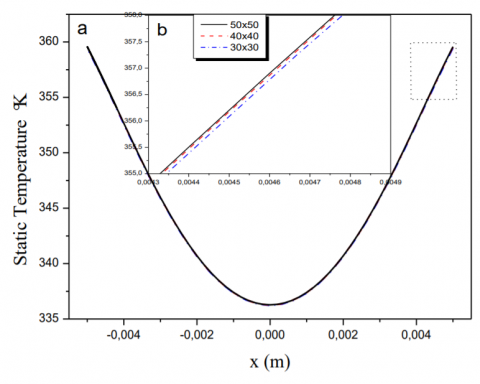Figure 2. Grid independence test: Static temperature, a) full profile, b) focusing on the selected zone

## 3.png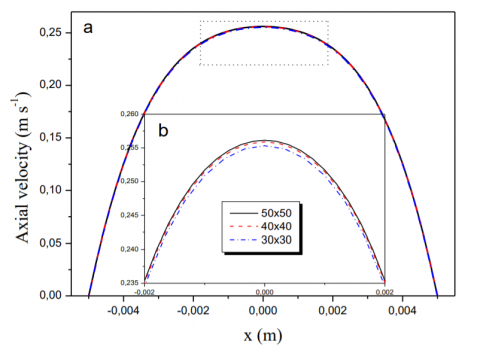Figure 3. Grid independence test: Axial velocity, a) full profile, b) focusing on the selected zone

## 4.pngFigure 4. Grid independence test: Nusselt number, a) full profile, b) focusing on the selected zone

A validation of the numerical model and the problem configuration was made before starting the numerical study. Table 1 refers to mixed convection regime with different values of the buoyancy parameter Ω: -100, 100, 200 and 300. A comparison between the values of the Nusselt number, the momentum flux correction factor Kd and the kinetic energy correction factor Ke acquired in the current paper and those obtained analytically by Barletta et al, 2003 is presented in Table 1. A very good agreement can be observed from the comparison between the present paper results and those obtained by Barletta et al, 2003, mainly for the low values of the buoyancy parameter Ω. A slight difference is found for the Nusselt number value, in the case of Ω=300, where the relative error between the numerical (present) and analytical results is 2.8%. This difference is maybe due to the use of the numerical methods, or to the choice of the reference temperature T0, where in the CFD computations is considered as constant and equal to the entrance temperature, whereas the reference temperature used in the analytical solution is the mean fluid temperature at each cross-section.

Table 1. Comparison between the values of Nu, Kd, and Ke obtained in the present work and those availaible in the leterature

 Ω Present paper                 Barletta et al.  Nu         Kd        Ke          Nu          Kd         Ke -100 2.728    1.546   2.791       2.743    1.547     2.795 100 3.420    1.266   1.762       3.423    1.273     1.778 200 3.740    1.200   1.546       3.630    1.204     1.550 300 4.040    1.161   1.424       4.156    1.171     1.449
4. Results and Discussions

In this section, the effects of the buoyancy parameter and Richardson number on the heat transfer level presented in terms of the Nusselt number and the flow velocity profile will be discussed. In order to achieve the mentioned goal, several simulations were performed during this study.

4.1 Thermal effect

Results are obtained for Re= 25, 50, 75 and 100. Thus, the inlet fluid temperature is considered equal to 300K, while the walls heat flux density q0 has been fixed to a constant value for all simulations. The working fluid is air and the Prandtl number is taken equal to 0.7. The Grashof number Gr and therefore Ω and Richardson number Ri, are then varied by varying the imposed heat flux density value q0 which is taken to range from -38.66 to 1546.58 w/m2. This gives a range of the Grashof number from -2.5 102 to 105 and consequently a ranges from -4 to 40 and from -102 to 103 of Ri and Ω respectively.

Figures 5, 6, 7 and 8 present the evolution of the local Nusselt number along the longitudinal coordinate z of the channel for various buoyancy parameters Ω equal to -100, 100, 200 and 300 respectively. For a given value of Ω, four values of the Richardson number were tested.

Figure 5 shows the development of the local Nusselt number with z coordinate for (Gr/Re) = -100. It is seen that the local Nusselt number is decreased with the z coordinate, with the asymptotic value equal to Nu= 2.728. It is also observed that, the same asymptotic value of the local Nusselt number is reached with different flow-establishment length for the different considered Richardson numbers. Moreover it is clearly visible that the flow-establishment length is declined with the increase of the Richardson number. For example with Ri= -1 the length of establishment is z= 8 cm, while it is equal to 2 cm when Ri= -4. So as to evaluate the parameters governing 3D laminar mixed convection flow in rectangular channels, the same experience is repeated with increasing the magnitude of the buoyancy parameter to Ω =100. Figure 6 illustrates the trends of the local Nusselt number calculated at the surfaces parallel to the entrance section along the z coordinate for Ω=100. An enhancement of the asymtotic local Nusselt number is observed compared to the earlier case, with the new constant local Nusselt number becomes Nu= 3.42. The flow-establishment length is also changed with the Richardson number, but with different lengths than the case of Ω=-100, for example at Ri = 4 the flow-establishment length is equal to z= 2 cm, and at Ri= 1 and Ri = 1.33 is 7 cm and 5 respectively.

## 5.png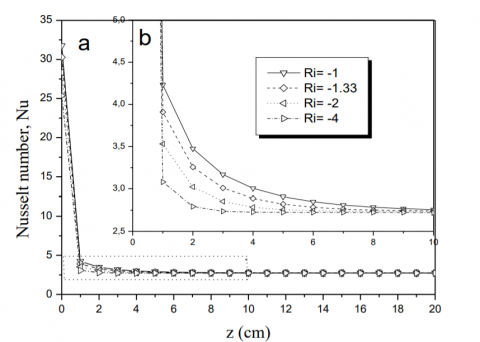Figure 5. Evolution of the local Nusselt number with the z coordinate for Ω=-100 and different values of Ri. a) Full profile, b) focusing on the selected zone

## 6.png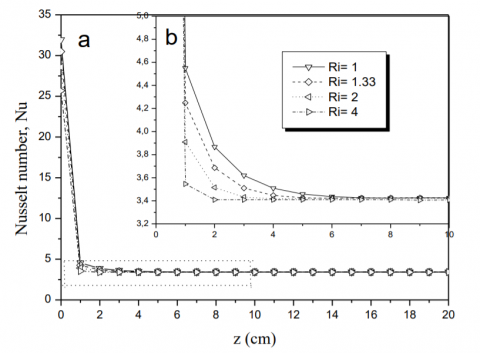Figure 6. Evolution of the local Nusselt number with z coordinate for Ω=100 and for different values of Ri. a) Full profile, b) focusing on the selected zone

Figure 7 demonstrates the effect of the buoyancy parameter Ω (=200) on the heat transfer level, where the asymptotic local Nusselt number increased from 2.72 and 3.42 in the previous two cases to 3.74 in the current case.

In figure 8, the previously found consequences are confirmed, where the constant local Nusselt number is improved with the augmentation of buoyancy parameter (Ω= 300) to Nu= 4.04. In addition the flow-establishment length is changed because of the variation of Ω and Ri. More specifically, the flow establishment length at Ri= 3, 4, 6 and 12 is respectively z= 4, 3, 2 and 1 cm.

We can declare that the obtained results with different values of Ω and Ri appears that the heat transfer rate (Nusselt number) in the 3D laminar mixed convection flow within a rectangular channel is not affected by the Richardson number parameter. Moreover the flow-establishment length is affected by the change of both the buoyancy parameter and the Richardson number.

## 7.png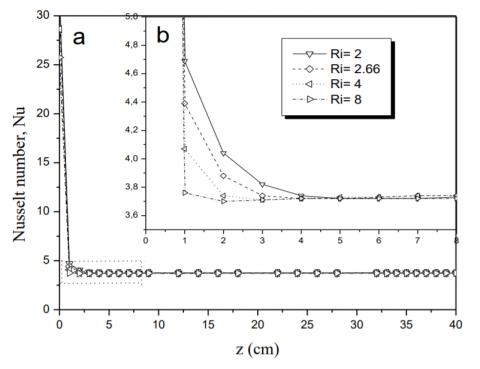Figure 7. Evolution of the local Nusselt number with the height of the duct (z), for Ω=200, and different values of Ri. a) Full profile, b) focusing on the selected zone

## 8.png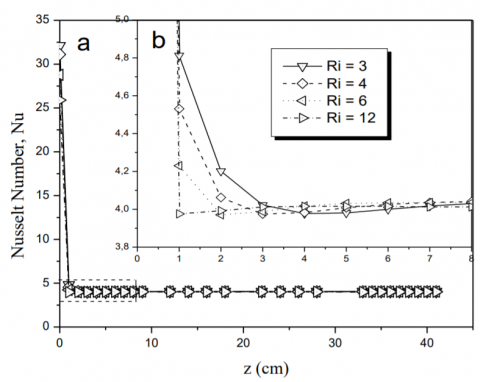Figure 8. Evolution of the local Nusselt number with the height of the duct (z), for Ω=300 and different values of Ri. a) Full profile, b) focusing on the selected zone

4.2 Dynamic effect

In order to examine the evolutions of the flow velocity profile with the Gr/Re ratio and Richardson number, Figure 9 illustrates the development of the axial velocity profiles with the channel length (x coordinate) at z=29 cm. The numerical simulations were realised for Re=100 and various values of the buoyancy parameter Ω (-100, 100, 200, 300, 400, 600, 800 and 1000).

For Ω =-100 and Ω =100 the velocity profiles are symmetric and parabolic with the maximum value is located at the centre of the duct. It should be noted that the two profiles of Ω -100 and 100 have the same values of the Graschof and Reynolds numbers, the unique difference between the two cases is that for Ω =-100Ω=-100 is a cooling of the fluid and Ω =100Ω=100 is a heating of the fluid. We can see that the fluid in the case of the cooling is more rapid in the centre region than the case of the heating. With Ω equal to 200, the velocity profile becomes almost flatness and the maximum value at the channel’s middle disappeared. Above Ω=200 and with the increase of the buoyancy parameter, the velocity profile is characterized by two maximums near the wall, and the flow becomes more slowly at the duct centre. As Ω increased to 1000, the two velocity symmetrical maximums shift away from the channel centre. Similar result of the axial velocity profile is found in the literature by Desrayaud and Lauriat. , for the dimensionless coordinate Z= 20 and Ω= 530, which is comparable to the obtained profile in the current paper for Z= 29 and Ω= 600.

## 9.png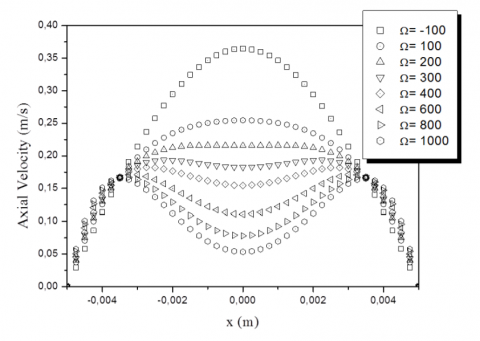Figure 9. Axial velocity profiles at the outlet cross section of the channel (z=39 cm) as a function of x coordinate for various values of Ω=Gr/Re, Re=100.

Figure 10, displays the axial velocity magnitude and pathlines in y-z plane for Ω= 1000 and Re= 100. Figure 10 upper panel illustrates the full channel, while the lower panel presents a focus on the outlet region; these two frames appear clearly the distribution of the velocity magnitude and the flow structure along the channel.

## 10.png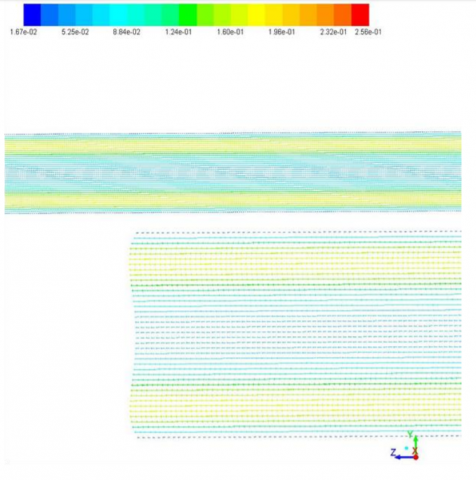Figure 10. Axial velocity magnitude and pathlines in y-z plane, full channel (upper panel), and outlet region (lower panel).  Re=100, Ω= 1000

Figure 11 presents the contour lines of the axial velocity distribution at the cross-section surface (z= 29 cm) for the selected values of the buoyancy parameter Ω, which is computed at the same Reynolds number Re = 100. Figure 11-a and b, shows that the velocity distribution for Ω = -100 and 100 has one maxima at the centre of the channel, and minimum values near the walls. Figure 11-c refers to Gr/Re= 200 and reveals the start of the appearance of four local maxima. Figures 11-d, e, f, g and h display the contour lines of the axial velocity for Ω= 300, 400, 600, 800 and 1000 respectively, these figures point out that the velocity distribution assumes always one local minimum value at the centre of the duct and four local maxima near the channel corners. An analysis of the figure 11 leads to the conclusion that; the increase of Ω= Gr/Re (with constant Reynolds number) decelerate the fluid flow near the channel centre and to accelerate the flow near the walls. The magnitude of the deformation of the velocity being dependent upon the Gr/Re ratio and the Richardson number Ri.

5. Conclusions

The aim of this work was to investigate numerically the parameters affecting 3D laminar and mixed convection flow regime inside a rectangular channel with imposed constant wall flux density. The nondimentional governing equations of continuity, momentum and energy are solved numerically, using a CFD code, and the Boussinesq approximation has been employed during this study. Obtained results of four different cases are compared to analytical study from the literature (Barletta et al. 2003). The comparison reveals a good agreement between our results and those obtained analytically.

In this paper, a parametric study was carried out to assess the influence of the buoyancy parameter (Gr/Re) and the Richardson number on the behaviour of the local Nusselt number, in addition to the fluid flow structure. More specifically, effects (thermal and dynamic) of the Gr/Re ratio and the Richardson number are examined. From one side; through the consideration of fixed value of Ω (-100, 100, 200 and 300) and changing the Richardson number Ri, with varying the Reynolds number Re (25, 50, 75 and 100). From the other side, the dynamic effect is assessed with fixed Reynolds number Re=100 and various values of the buoyancy number Ω (-100, 100, 200, 300, 400, 600, 800 and 1000) which obtained by varying the magnitude of the heat flux density.

The local Nusselt number computed at each cross-section increases with increasing the buoyancy number Ω, which clears up the effect of the ratio Gr/Re on the heat transfer rate. On the other hand, while increasing the Richardson number within the same buoyancy parameter value (fixed Ω), the computed local Nusselt number is kept constant. This result demonstrates that the main parameter characterising the mixed convection regime is the ratio Gr/Re instead of the Richardson number. Besides, it is spotted that the flow-establishment length is well affected by both; the buoyancy constant and the Richardson number.

Due to the effect of buoyancy number Ω and Richardson number Ri, fluid flow accelerated near the hot walls and decelerated at the centreline of the duct. The magnitude of the deformation of the velocity being dependent upon the buoyancy number Gr/Re and the Richardson number Ri.

## 11a.png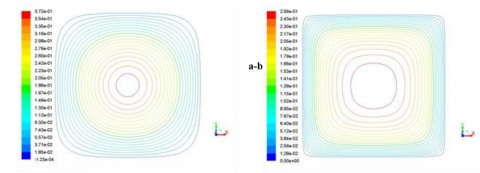## 11b.png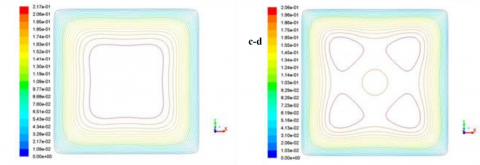## 11c.png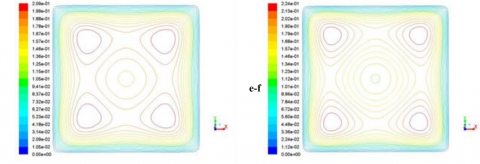## 11d.png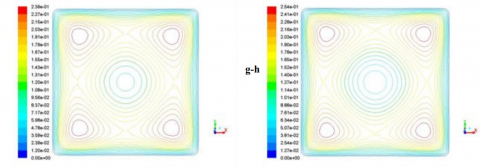Figure 11. Contour lines of axial velocity at the cross section plane (z= 39 cm) for various values of Gr/Re ratio and at fixed Reynolds number. Re = 100. a) Ω= -100. b) Ω= 100. c) Ω= 200. d) Ω= 300. e) Ω= 400. f) Ω= 600. g) Ω= 800. h) Ω= 1000.

Nomenclature
 a width of the rectangular cross-section, m Cp specific heat of the fluid, J. kg-1. K-1 D hydraulic diameter, m g Gr gravitational acceleration, m.s-2 Grashof number h average convection coefficient W.m-2.K-1 Kd momentum flux correction factor, defined by eq: (8) Ke kinetic energy correction factor, defined by eq: (9) Nu Nusselt number P pressure, Pa Pr Prantdl number q0 wall heat flux density, W.m-2 Re Reynolds number S Cross-section surface, m2 T temperature, K T0 the reference temperature u z z-component of the fluid velocity, m. s-1 u z, 0 mean fluid velocity in a duct cross-section, m. s-1 U dimensionless velocity vector X, Y, Z dimensionless coordinate system Greek symbols β thermal expansion coefficient, K-1 θ dimensionless temperature λ thermal conductivity of the fluid, W. m-1. K-1) µ dynamic viscosity, kg. m-1.s-1 ν kinematic viscosity of fluid, m2.s-1 ρ density of the fluid, kg/m3 ρ0 density of the fluid for T= T0, kg.m-3 Ω buoyancy parameter, Ω= Gr/Re Subscripts ∞ free-stream x, y, z cartesian coordinates w wall
References

 Mohammed, H. A., “Laminar mixed convection heat transfer in a vertical circular tube under buoyancy-assisted and opposed flows,” Energy Conversion and Management., vol. 49, no. 8, pp. 2006-2015, 2008. DOI: 10.1016/j.enconman.2008.02.009.

 Zeghbil, I., Bessaih, R., “Mixed convection in lid-driven cavities filled with a nanofluid,” International Journal of Heat and Technology., vol. 33, no. 4, pp. 77-84, 2015. DOI: 10.18280/ijht.330410.

 Dawood, H. K., Mohammed, H. A., Che Sidik, N. A., Munisamy, K. M., Wahid, M. A., “Forced, natural and mixed-convection heat transfer and fluid flow in annulus: A review,” International Communications in Heat and Mass Transfer., vol. 62, pp. 45-57, 2015.  DOI: 10.1016/j.icheatmasstransfer.2015.01.006.

 Koffi, F., Abid, C., Medale, M., Papini, F., “Mixed convection flow in a horizontal rectangular channel subjected to a horizontal thermal gradient,” Heat Mass Transfer., vol. 47, pp. 251–1260, 2011. DOI: 10.1007/s00231-011-0775-y.

 Elliott, L., Ingham, D. B., and Wood, J. D., “Mixed Convection Flow of Newtonian and Non-Newtonian Fluids in a Horizontal Rectangular Duct,” Numerical Heat Transfer Part A., vol. 32, pp. 831-860, 1997.  DOI: org/10.1080/10407789708913920.

 Barletta, A., Rossi di Schio, E., Zanchini, E., “Combined forced and free flow in a vertical rectangular duct with prescribed wall heat flux,” International Journal of Heat and Fluid Flow., vol. 24 pp. 874-887, 2003. DOI: 10.1016/S0142-727X(03)00090-0.

 Mohammed, H. A., Nur Imrawati Om, Shuaib, N. H., Hussein, A. K., Saidur, R., “The application of nanofluids on three dimensional mixed convection heat transfer in equilateral triangular duct,” International Journal of Heat and Technology., vol. 29, no. 2, pp. 3-12, 2011.

 Yang, G., Wu, J. Y., Yan, L., “Flow reversal and entropy generation due to buoyancy assisted mixed convection in the entrance region of a three dimensional vertical rectangular duct,” International Journal of Heat and Mass Transfer., vol. 67, pp. 741-751, 2013. DOI: 10.1016/j.ijheatmasstransfer.2013.08.089.

 Yang, G., Wu, J. Y., “Effect of aspect ratio and assisted buoyancy on flow reversal for mixed convection with imposed flow rate in a vertical three dimensional rectangular duct,” International Journal of Heat and Mass Transfer., vol. 77, pp. 335-343, 2014. DOI: 10.1016/j.ijheatmasstransfer.2014.05.027.

 Kurtbas, I., Celik, N., “Experimental investigation of forced and mixed convection heat transfer in a foam-filled horizontal rectangular channel,” International Journal of Heat and Mass Transfer., vol. 52, pp. 1313-1325, 2009. DOI: 10.1016/j.ijheatmasstransfer.2008.07.050.

 Yang, M. H., Yeh, R. H., Hwang, J. J., “Mixed convective cooling of a fin in a channel,” International Journal of Heat and Mass Transfer., vol. 53, pp. 760-771, 2010. DOI: 10.1016/j.ijheatmasstransfer.2009.10.012.

 Fu, W. S., Lai, Y. C., Huang, Y., Liu, K. L., “An investigation of flow reversal of mixed convection in a three dimensional rectangular channel with a finite length,” International Journal of Heat and Mass Transfer., vol. 64 pp. 636-646, 2013. DOI: 10.1016/j.ijheatmasstransfer.2013.04.025.

 Fu, W. S., Chao, W. S., Wu, P. J., Lin, Y. M., “A new method of adjustment of inlet boundary for improving heat transfer of mixed convection in a vertical channel,” International Communications in Heat and Mass Transfer., vol. 77 pp. 165–173, 2016. DOI: 10.1016/j.icheatmasstransfer.2016.08.002.

 Marroquin, J., Trevino, C., Cajas, J. C., Salcedo, E., Martinez-Suastegui, L., “Numerical study on buoyancy and inclination effects on transient laminar opposing mixed convection in rectangular channels with symmetric and discrete heating,” International Journal of Heat and Mass Transfer., vol. 84 pp. 766-785, 2015. DOI: 10.1016/j.ijheatmasstransfer.2014.12.080.

 Ichimiya, K., Matsushima, Y., “Performance evaluation of mixed convection in an inclined square channel with uniform temperature walls,” International Journal of Heat and Mass Transfer., vol. 52, pp. 1802-1810, 2009. DOI: org/10.1016/j.ijheatmasstransfer.2008.10.016.

 Fakour, M., Vahabzadeh, A., Ganji, D. D., “Scrutiny of mixed convection flow of a nanofluid in a vertical channel,” Case Studies in Thermal Engineering., vol. 4, pp. 15-23, 2014. DOI: 10.1016/j.csite.2014.05.003.

 Yang, G., Wu, J. Y., “Conjugate mixed convection in the entrance region of a symmetrically heated vertical channel with thick walls,” International Journal of Thermal Sciences., vol. 98, pp. 245-254, 2015. DOI: 10.1016/j.ijthermalsci.2015.07.023.

 Barletta, A., Zanchini, E., “On the choice of the reference temperature for fully-developed mixed convection in a vertical channel,” Int. J. Heat Mass Transfer., vol. 42, pp. 3169-3181, 1999. DOI: org/10.1016/S0017-9310(99)00011-3.

 Barletta, A., Lazzari, S., Magyari, E., Pop, I., “Mixed convection with heating effects in a vertical porous annulus with a radially varying magnetic field,” International Journal of Heat and Mass Transfer., vol. 51 pp. 5777-5784, 2008. DOI: org/10.1016/j.ijheatmasstransfer.2008.05.018.

 Zanchini, E., “Effect of viscous dissipation on mixed convection in a vertical channel with boundary conditions of the third kind,” International Journal of Heat and Mass Transfer., vol. 41, pp. 3949-3959, 1998. DOI: 10.1016/S0017-9310(98)00114-8.

 Umavathia, J. C., Sheremet, M. A., “Mixed convection flow of an electrically conducting fluid in a vertical channel using Robin boundary conditions with heat source/sink,” European Journal of Mechanics B/Fluids., vol. 55, pp, 132-145, 2015. DOI: 10.1016/j.euromechflu.2015.08.013.

 Shah, R. K., London, A. L., “Laminar forced convection in ducts,” In: Irvine, T.F., Hartnett, J.P. (Eds.), Advances in Heat Transfer. Academic Press, New York.

 Desrayaud, G., Lauriat, G., “Flow reversal of laminar mixed convection in the entry region of symmetrically heated, vertical plate channels,” International Journal of Thermal Sciences., vol. 48, pp. 2036–2045, 2009. DOI: 10.1016/j.ijthermalsci.2009.03.002.